upd@2022/9/14：最近把实验的代码放到 github 上了，如果需要参考可以查看这里：

https://github.com/ttzytt/xv6-riscv

int
main(int argc, char* argv[]){
}

# Lab 1: utils

## sleep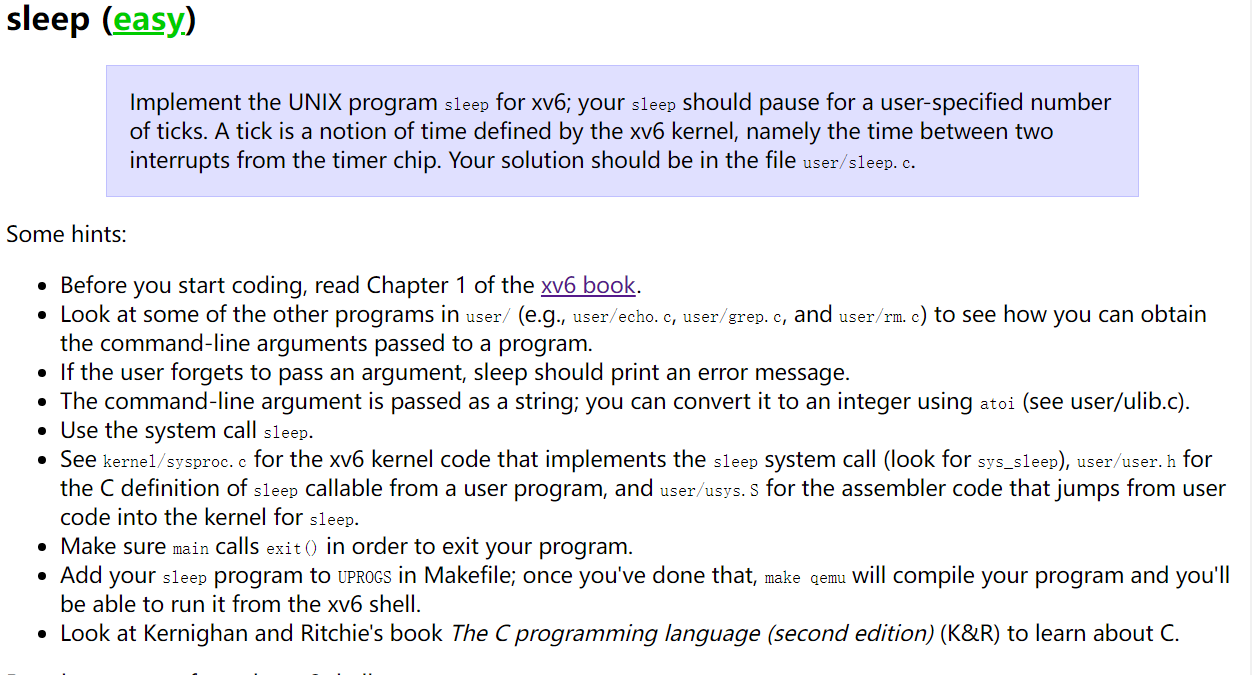#include "kernel/types.h"
#include "kernel/stat.h"
#include "user/user.h"
#include "kernel/fd_types.h"

int main(int argc, char *argv[]){
if (argc != 2){
fprintf(STDERR, "usage: sleep <tick count>");
exit(1);
}
int tm = atoi(argv); // 字符串 -> 整数
sleep(tm);
exit(0);
}   

#pragma once
const char STDIN  = 0;
const char STDOUT = 1;
const char STDERR = 2;

## pingpong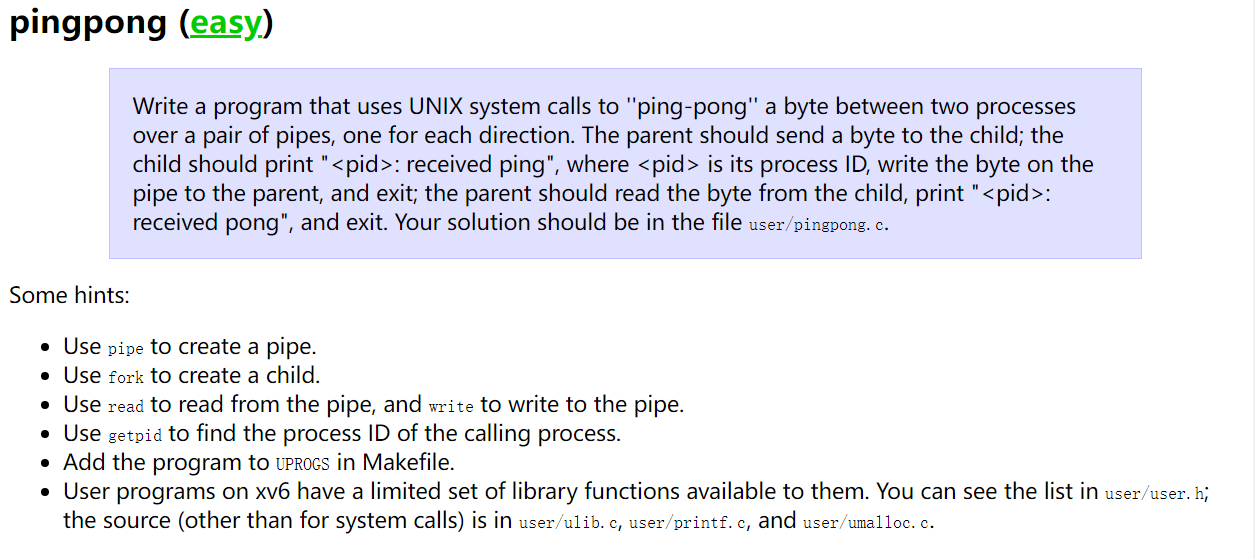#include "kernel/fd_types.h"
#include "kernel/types.h"
#include "user/user.h"
enum PIPE_END { REC = 0, SND = 1 };

int main(int argc, char* argv[]) {
if (argc != 1) {
fprintf(STDERR, "usage: pingpong (no parameter)");
exit(114514);  //（悲
}
int p;
pipe(p);
int cur_pid = fork();

if (cur_pid == 0) {
//子进程
//子进程先接收消息
char buf;
if (read(p[REC], buf, sizeof(buf)) > 0) {
}
//子进程用管道发送消息给父进程
fprintf(p[SND], "child");
exit(0);
} else if (cur_pid > 0) {
char buf;

//父进程先发送消息，后接收消息
fprintf(p[SND], "parent");
wait(0);
}
exit(0);
} else {
fprintf(STDERR, "failed to fork");
exit(1919810);// homo 特有的 exit 参数（悲
}
exit(0);
}

## primes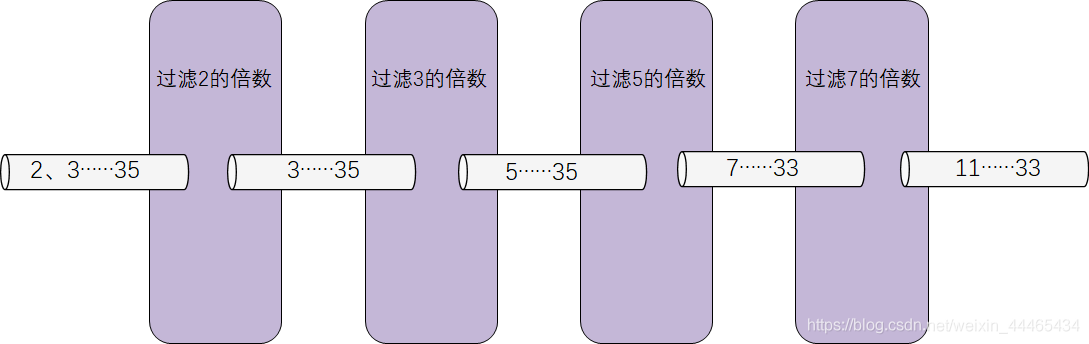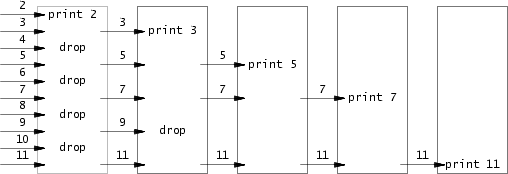int main(int argc, char* argv[]) {
int pp;
pipe(pp);
int pid;
pid = fork();
if (pid == 0) {
close(pp[SND]);
child_proc(pp);
} else {
int init_num[MAX_P];
int idx = 0;
for(int i = 2; i <= MAX_P; i++){
init_num[idx++] = i;
}
close(pp[REC]);
send_to_next(pp[SND], init_num, idx);
close(pp[SND]);
wait(0);
}
exit(0);
}

void send_to_next(int outpp, int msg[], int msg_len) {
//发送到下一个子进程
//outpp 是管道的发送端
for (int i = 0; i < msg_len; i++) {
write(outpp, msg + i, sizeof(int));
}
}

void child_proc(int pp) {
int child_pp;
pipe(child_pp);
int prime;
int len = read(pp[REC], &prime, sizeof(int));

if(len == 0){
//如果全部都筛掉了，那自然可以结束了
printf("OK");
exit(0);
return;
}
printf("prime %d\n", prime);
int outlen;
int* filtered = filter(prime, pp[REC], &outlen);
close(pp[REC]);

int pid = fork();
if(pid == 0){
close(child_pp[SND]);
child_proc(child_pp);
} else {
close(child_pp[REC]);
send_to_next(child_pp[SND], filtered, outlen);
close(child_pp[SND]);
wait(0); // wait 可以释放子进程的进程号以及别的资源
exit(0);
}
}

int* filter(int num, int inpp, int* outlen) {
//把 inpp 管道中的 num 倍数全部过滤掉，返回过滤好的数组（没有 num 的倍数）
(*outlen) = 0;
//len 是过滤之后还有多少个数字
int* out = (int *)malloc(MAX_P * sizeof(int));
int ret = 0;
do {
ret = read(inpp, out + (*outlen), sizeof(int));
// ret 返回读到了多少字节
if (out[(*outlen)] % num != 0 && ret > 0) {
(*outlen)++;
}
} while (ret > 0);
return out;
}   

if(pid == 0){
close(child_pp[SND]);
child_proc(child_pp);
}

read() 一个管道时，如果不 close() 这个管道的发送端，这个 read() 是会阻塞的，也就是会卡在这里，等待新数据。因为系统不知道之后会不会有信息从发送端发过来。只有关闭了发送端才能表明传输已经结束，之后再也不会有新的数据从发送端传过来。#include "kernel/fd_types.h"
#include "kernel/types.h"
#include "user/user.h"
#include "kernel/dbg_macros.h"
const int MAX_P = 35;
// #define FDEBUG
enum PIPE_END { REC = 0, SND = 1 };
void send_to_next(int outpp, int msg[], int msg_len) {
//发送到下一个子进程
for (int i = 0; i < msg_len; i++) {
write(outpp, msg + i, sizeof(int));
}
}
int* filter(int num, int inpp, int* outlen) {
//把 inpp 管道中的 num 倍数全部过滤掉，返回过滤好的数组（没有 num 的倍数）
(*outlen) = 0;
//len 是过滤之后还有多少个数字
int* out = (int *)malloc(MAX_P * sizeof(int));
int ret = 0;
do {
ret = read(inpp, out + (*outlen), sizeof(int));
// ret 返回读到了多少字节
if (out[(*outlen)] % num != 0 && ret > 0) {
(*outlen)++;
}
} while (ret > 0);
return out;
}

void child_proc(int pp) {
int child_pp;
pipe(child_pp);
int prime;
int len = read(pp[REC], &prime, sizeof(int));
DEBUG("len: %d\n", len);
if(len == 0){
printf("OK");
exit(0);
return;
}
printf("prime %d\n", prime);
int outlen;
int* filtered = filter(prime, pp[REC], &outlen);
dbg_arr_i32(filtered, 0, outlen);
DEBUG("outlen: %d\n", outlen);
close(pp[REC]);

int pid = fork();
if(pid == 0){
close(child_pp[SND]);
child_proc(child_pp);
} else {
close(child_pp[REC]);
send_to_next(child_pp[SND], filtered, outlen);
close(child_pp[SND]);
wait(0); // wait 可以释放子进程的进程号以及别的资源
exit(0);
}
}

int main(int argc, char* argv[]) {
int pp;
pipe(pp);
int pid;
pid = fork();
if (pid == 0) {
close(pp[SND]);
child_proc(pp);
} else {
int init_num[MAX_P];
int idx = 0;
for(int i = 2; i <= MAX_P; i++){
init_num[idx++] = i;
}
close(pp[REC]);
send_to_next(pp[SND], init_num, idx);
close(pp[SND]);
wait(0);
}
exit(0);
}

#pragma once
#include "kernel/fd_types.h"

#if (!defined FPRINTF)
//内核态是没有定义 fprintf 的，只有 printf，所以重新定义 printf
#define fprintf(_stream, _fmt, ...) printf(_fmt, ##__VA_ARGS__)
#endif

#ifdef FDEBUG
#define try(_expr, _act)                                                     \
{                                                                        \
if ((_expr) < 0) {                                                   \
fprintf(STDERR, "try: %s failed, at line %d, file %s\n", #_expr, \
__LINE__, __FILE__);                                     \
_act;                                                            \
}                                                                    \
}
#else
#define try(_expr, _act)
#endif

#ifdef FDEBUG
#define DEBUG(fmt, ...) fprintf(STDERR, fmt, ##__VA_ARGS__)
#else
#define DEBUG(fmt, ...)
#endif

void dbg_arr_i32(int arr[], int st, int ed) {
#ifdef FDEBUG
for (int i = st; i <= ed; i++) {
DEBUG("%d ", arr[i]);
}
DEBUG("\n");
#endif
}

## find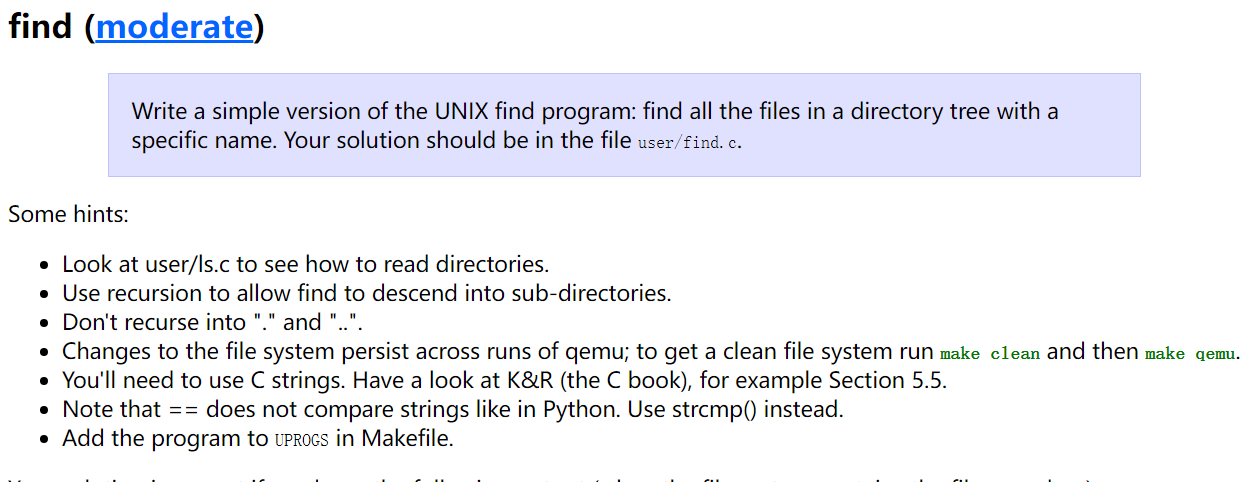struct dirent {
ushort inum;
char name[DIRSIZ];
};

ls 中，因为不是递归实现的，所以对于最开始的文件节点需要调用 fstat() 来判断是文件夹还是文件。然后如果是文件夹，再调用 stat() 来输出该文件夹内每个文件节点的信息。

stat()fstat() 都是用来获取文件节点信息的，唯一的不同是，fstat() 接收的是这个文件的标识符，而 stat() 则接收路径。

#include "kernel/fd_types.h"
#include "kernel/types.h"
#include "kernel/fs.h"
#include "kernel/stat.h"
#include "user/user.h"
// #define FDEBUG
#include "kernel/dbg_macros.h"

const int BUF_SIZ = 512;

char* get_fname_from_path(char path[]) {
char* ptr = path + strlen(path);  // ptr 指向 path 的最后一个元素
for (; ptr >= path && *ptr != '/'; ptr--) {
}
return ++ptr;  // 从 for 里出来指向的是 '/'，所以要减一下
}

void dfs_find(char* cur_path, char* name) {
int cur_fd;
char nexdir_buf[BUF_SIZ];
struct stat cur_stat;
struct dirent nex_dir;
try(cur_fd = open(cur_path, 0), return );
try(fstat(cur_fd, &cur_stat), return );  // fstat 接收的是一个文件描述符
if (cur_stat.type == T_FILE) {
if (strcmp(get_fname_from_path(cur_path), name) == 0) {
printf("%s\n", cur_path);
}
} else if (cur_stat.type == T_DIR) {
strcpy(nexdir_buf, cur_path);
char* path_end = nexdir_buf + strlen(nexdir_buf);

*(path_end) = '/';
path_end++;
while (read(cur_fd, &nex_dir, sizeof(struct dirent)) ==
sizeof(struct dirent)) {
if (nex_dir.inum == 0)
continue;  // inum 就是文件节点，等于 0 为不可用
if (strcmp(".", nex_dir.name) == 0 || strcmp("..", nex_dir.name) == 0){
DEBUG(". or ..\n");
continue;
}
memmove(path_end, nex_dir.name, DIRSIZ);
path_end[DIRSIZ] = '\0';
try(stat(nexdir_buf, &cur_stat),
continue);  //这里的 stat 接收的是绝对路径，并且这句话是可以删掉的，因为是递归实现。
dfs_find(nexdir_buf, name);
}
}
close(cur_fd);
}

int main(int argc, char* argv[]) {
if (argc != 3) {
fprintf(STDERR, "usage: find <directory> <file name>");
exit(114);
}
dfs_find(argv, argv);
exit(0);
}

## xargs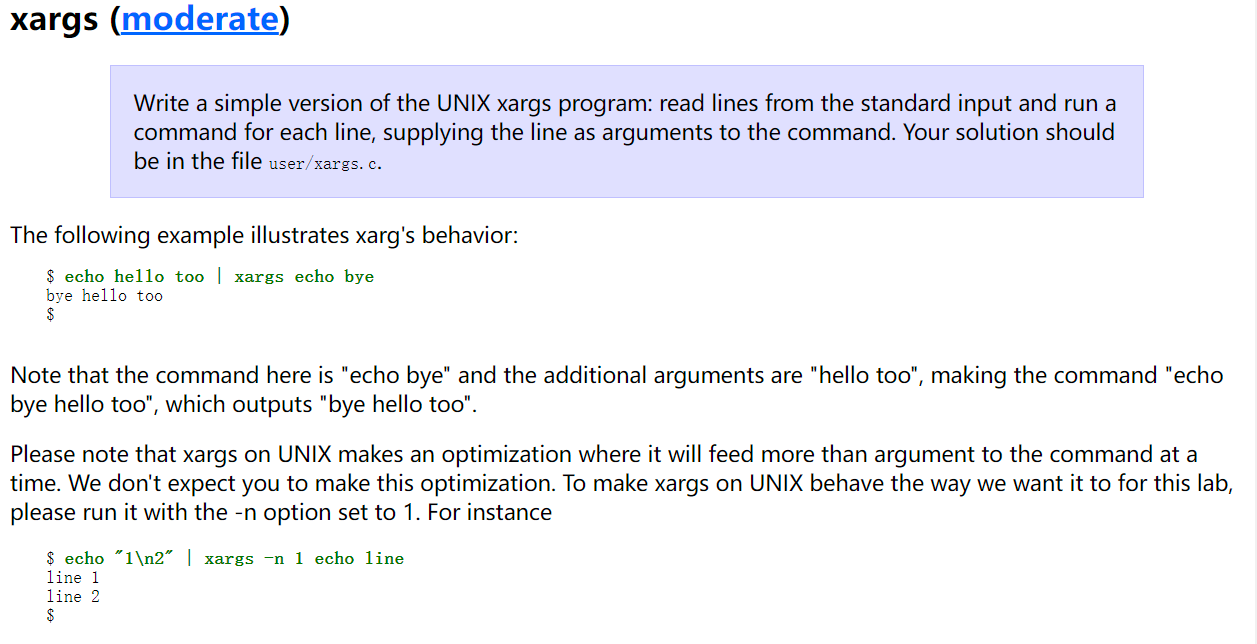#include "kernel/types.h"
#include "user/user.h"
// #define FDEBUG
#include "kernel/fd_types.h"
#include "kernel/param.h"
#include "kernel/dbg_macros.h"

const char* DEFAULT_CMD = "echo";
#define MX_ARG_CNT 32
#define MX_ARG_LEN 32

char cut_str_by(char* src, char* dst, int* srcpos, char* signs) {
// 从 src 串的下标为 srcpos 的位置开始往后找，在第一个碰到 signs 里面的字符时停下来
// 然后把 src[srcpos……<碰到 sign 的位置>] 这段字符串截取下来，放入 dst 中。
// 注意这个 srcpos 是一个指针，也就是调用完这个函数后我们可以通过 srcpos 知道在哪里
// 碰到了 signs
// 返回值其实是一个布尔类，但是因为 c 语言没有，就用了 char，其表示是否碰到 signs 里
// 的字符
// 如果没有的话可能是 srcpos 这个位置就是一个 \0，也就是没有新的参数了。也可能是读完
// 了一段字符，后面没空格和 \n 了，那么说明这是最后一个参数。
for (int i = *srcpos; src[i] != '\0'; i++) {
for (int s = 0; signs[s] != '\0'; s++) {
if (src[i] == signs[s]) {
src[i] = '\0';
strcpy(dst, src + *srcpos);
*srcpos = i + 1;
return 1;
}
}
}
return 0;
};

char std_args[MX_ARG_CNT][MX_ARG_LEN];
int main(int argc, char* argv[]) {
char* cmd;
if (argc == 1) {
cmd = DEFAULT_CMD;
} else {
cmd = argv;
}

int argcnt = 0;
char buf[MX_ARG_LEN * MX_ARG_CNT];
int curlen = 0;
int lst_pos = 0;

memset(std_args, 0, sizeof(std_args));
while (cut_str_by(buf, std_args[argcnt], &lst_pos, "\n ")) {
while (buf[lst_pos] == '\n' || buf[lst_pos] == ' ') {
//可能两个参数之间隔了很多个空格
lst_pos++;
}
argcnt++;
}

char* arg2pass[MX_ARG_CNT];

int lst = 0;
arg2pass[lst++] = cmd; // 先放 argv
for (int i = 2; i < argc; i++) {
// 然后是其他 argv
arg2pass[lst++] = argv[i];
}
for (int i = 0; i < argcnt; i++) {
// 最后放从标准输入来的 argv
arg2pass[lst++] = std_args[i];
}

exec(cmd, arg2pass);
exit(0);
}

## 总结